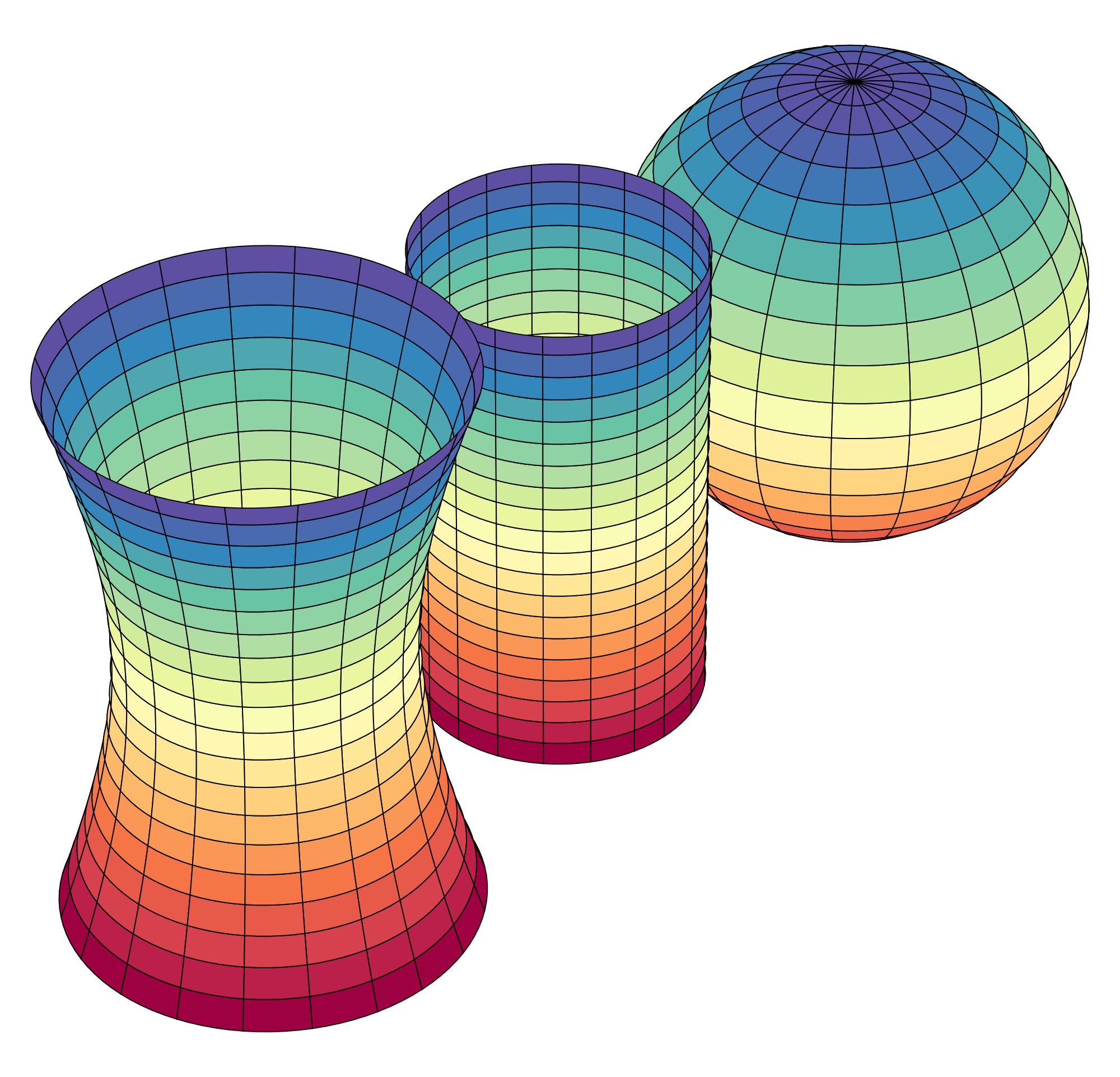# Multivariable Calculus - After the AP Calculus AB and BC Courses

An excellent path towards academic achievement in university calculus is to take the Multivariable Calculus course after you have completed the high-school AP Calculus AB and BC courses.

####Upper Division Math Courses

Some students pass the AP Calculus AB and/or BC exams at a 3, 4, or 5, earning college credit at their college or university they will attend after high school.

Keep the ball rolling! Many motivated high school students take the Distance Calculus Multivariable Calculus course after they complete the AP Calculus AB and BC course at their high school.

Distance Calculus does not require a specific score on the AP Calculus BC exam - completion of your high school AP Calculus BC course is sufficient to meet the prerequisite for the Distance Calculus Multivariable Calculus course. Although earning course credit for Calculus I (Calculus AB) and/or Calculus II (Calculus BC) is advantageous for an eager high school student, sometimes the high stakes AP Calculus exam is not for everyone. It is more important to keep your math studies going forward, and Distance Calculus Multivariable Calculus can be your platform to earn real, transferrable college credits, not just a score on a standardized exam.

Here is a video on the AP Calculus exams and our Multivariable Calculus course:

## Distance Calculus - Student ReviewsDate Posted: Feb 23, 2020
Review by: Carl Conners
Courses Completed: Multivariable Calculus, Differential Equations, Linear Algebra
Review: After a really rough first year of calculus, I completed all of the second year calculus courses with Distance Calculus. It was like night and day the difference. My first year was so boring and monotonous. Multivariable Calculus, Differential Equations, and Linear Algebra through Distance Calculus were just so much different - so not boring at all. I thoroughly enjoyed these courses. So engaging.
Transferred Credits to: Michigan State UniversityDate Posted: Jul 25, 2020
Review by: Michael Linton
Student Email: mdl264@cornell.edu
Courses Completed: Calculus I
Review: Amazing professor, extremely helpful and graded assignments quickly. To any Cornellians out there, this is the Calculus Course to take in Summer to fulfill your reqs! I would definitely take more Calculus Classes this way in the future!
Transferred Credits to: Cornell UniversityDate Posted: Jan 19, 2020
Review by: William Williams
Student Email: wf.williamster@gmail.com
Courses Completed: Linear Algebra, Probability Theory
Review: I have difficulty learning calculus based math, akin to dyslexia when examining the symbolic forms, equations, definitions, and problems. Mathematica based calculus courses allowed me to continue with my studies because of the option of seeing the math expressed as a programming language for which I have no difficulty in interpreting visually and the immediate feedback of graphical representations of functions, equations, or data makes a huge impact on understanding. Mathematica based calculus courses should be the default method of teaching Calculus everywhere.
Transferred Credits to: Thomas Edison State College